# High Precision Orbit Propagation of the International Space Station

This example shows how to propagate the orbit of the International Space Station (ISS) using high precision numerical orbit propagation with Aerospace Blockset™. It uses the Orbit Propagator block to calculate the ISS trajectory for 24 hours. The example then compares position and velocity states to publicly available ISS trajectory data available from NASA Trajectory Operations and Planning (TOPO) flight controllers at Johnson Space Center.

The Orbit Propagator block models translational dynamics of spacecraft using numerical integration. It computes the position and velocity of one or more spacecraft over time. For the most accurate results, use a variable step solver with low tolerance settings (less than 1e-9). Depending on your mission requirements, you can increase speed by using larger tolerances. Doing so might impact the accuracy of the solution. To propagate orbital states, the block uses the gravity model selected for the current central body. The block also includes atmospheric drag (for Earth orbits), and external perturbing accelerations provided as inputs to the block.

In this example, use ISS International Celestial Reference Frame (ICRF) position and velocity states directly from the referenced NASA public distribution file  to initialize an ISS orbit in the Orbit Propagator block. Use the EGM2008 spherical harmonic gravity model to model Earth's gravity, and the NRLMSISE-00 atmospheric model to compute atmospheric drag. Calculate third body gravity perturbations (for the Moon, Sun, and other planets in the solar system) and solar radiation pressure (SRP) outside of the Orbit Propagator block and pass the results into the block as external applied accelerations. Run the model for 24 hours, and compare against the published NASA trajectory data.

### Set Mission Initial Conditions

This example uses MATLAB® structures to organize mission data. These structures make accessing data later in the example more intuitive. They also help declutter the global base workspace.

The referenced ISS ephemeris data file  provided by NASA begins on January 3, 2022 at 12:00:00.000 UTC.

```mission.StartDate = datetime(2022, 1, 3, 12, 0, 0); mission.Duration = hours(24);```

The file contains ICRF position (km) and velocity (km/s) data sampled every 4 minutes.

```iss.X0_icrf = [-1325.896391725290 5492.890955896010 3762.423747679220]; % km iss.V0_icrf = [-4.87470128630892 -4.10251688094599 4.26428812476909]; % km/s```

It also contains mass (kg), drag area (m^2), and drag coefficient data corresponding with the epoch.

```iss.Mass = 459023.0; % kg iss.DragArea = 1951.0; % m^2 iss.DragCoeff = 2.0;```

The file also includes solar radiation area (m^2) and a solar radiation coefficient; however these values are zero because SRP is not prominent in low Earth orbit (LEO) where the ISS operates. Despite their trivial impact on the resultant trajectory, we will include solar radiation pressure calculations in this example to fully demonstrate high precision orbit propagation. SRP is more prominent at higher orbital regimes.

```iss.SRPArea = 1500; % m^2 iss.SRPCoeff = 1.8; iss.P_sr = 4.5344321e-6; % N/m^2```

### High Precision Orbit Propagation Algorithm

This example performs high precision numerical orbit propagation using Cowell's method. In the inertial (ICRF) frame, Earth's gravitational acceleration is summed with all acceleration perturbations and double-integrated to find velocity and position at each time step of the simulation.

`${\stackrel{\to }{\mathit{a}}}_{\mathrm{icrf}}={\stackrel{\to }{\mathit{a}}}_{\mathrm{gravity}}+{\stackrel{\to }{\mathit{a}}}_{\mathrm{drag}}+{\stackrel{\to }{\mathit{a}}}_{3\mathrm{rd}\text{\hspace{0.17em}}\mathrm{body}}+{\stackrel{\to }{\mathit{a}}}_{\mathrm{srp}}$`

`${\stackrel{\to }{\mathit{a}}}_{\mathrm{icrf}}\underset{\mathrm{integrate}}{⟹}{\stackrel{\to }{\mathit{v}}}_{\mathrm{icrf}},{\stackrel{\to }{\mathit{r}}}_{\mathrm{icrf}}$`

where:

${\stackrel{\to }{\mathit{a}}}_{\mathrm{gravity}}$ is the acceleration due to Earth gravity.

${\stackrel{\to }{\mathit{a}}}_{\mathrm{drag}}$ is the acceleration due to atmospheric drag.

${\stackrel{\to }{\mathit{a}}}_{3\mathrm{rd}\text{\hspace{0.17em}}\mathrm{body}}={\stackrel{\to }{\mathit{a}}}_{\mathrm{Moon}}+{\stackrel{\to }{\mathit{a}}}_{\mathrm{Sun}}+{\stackrel{\to }{\mathit{a}}}_{\mathrm{Mercury}}+{\stackrel{\to }{\mathit{a}}}_{\mathrm{Venus}}+{\stackrel{\to }{\mathit{a}}}_{\mathrm{Mars}}+{\stackrel{\to }{\mathit{a}}}_{\mathrm{Jupiter}}+{\stackrel{\to }{\mathit{a}}}_{\mathrm{Saturn}}+{\stackrel{\to }{\mathit{a}}}_{\mathrm{Uranus}}+{\stackrel{\to }{\mathit{a}}}_{\mathrm{Neptune}}$ is the acceleration due to gravity of the Moon, Sun, and planets of the solar system.

${\stackrel{\to }{\mathit{a}}}_{\mathrm{srp}}$ is the acceleration due to solar radiation pressure.

#### Earth Gravity

The gravity model for Earth in this example is the EGM2008 spherical harmonic gravity model. This model accounts for zonal, sectoral, and tesseral harmonics. For reference, the second-degree zeroth order zonal harmonic ${\mathit{J}}_{2}$, which accounts for the oblateness of Earth, is $-{\mathit{C}}_{2,0}$. Spherical Harmonic models accounts for harmonics up to max degree $\mathit{l}={\mathit{l}}_{\mathrm{max}}$. For EGM2008, ${\mathit{l}}_{\mathrm{max}}=2159$ .

Spherical harmonic gravity is computed in the fixed frame (ff) coordinated system (International Terrestrial Reference Frame (ITRF), in the case of Earth). Numerical integration, however, is always performed in the inertial ICRF coordinate system. Therefore, at each timestep, position and velocity states are transformed into the fixed-frame, gravity is calculated in the fixed-frame, and the resulting acceleration is transformed into the inertial frame.

`${\stackrel{\to }{\mathit{a}}}_{\mathrm{gravity}}=-\frac{\mu }{{\mathit{r}}^{3}}\stackrel{\to }{{\mathit{r}}_{\mathrm{icrf}}}+\mathrm{ff2icrf}\left({\stackrel{\to }{\mathit{a}}}_{\mathrm{nonspherical}}\right)$`

where:

`$\begin{array}{l}{\stackrel{\to }{\mathit{a}}}_{\mathrm{nonspherical}}=\left\{\left[\frac{1}{\mathit{r}}\frac{\partial }{\partial \mathit{r}}\mathit{U}-\frac{{{\mathit{r}}_{\mathrm{ff}}}_{\mathit{k}}}{{\mathit{r}}^{2}\sqrt{{{{\mathit{r}}_{\mathrm{ff}}}_{\mathit{i}}}^{2}+{{{\mathit{r}}_{\mathrm{ff}}}_{\mathit{j}}}^{2}}}\frac{\partial }{\partial \varphi }\mathit{U}\right]{{\mathit{r}}_{\mathrm{ff}}}_{\mathit{i}}-\left[\frac{1}{{{{\mathit{r}}_{\mathrm{ff}}}_{\mathit{i}}}^{2}+{{{\mathit{r}}_{\mathrm{ff}}}_{\mathit{j}}}^{2}}\frac{\partial }{\partial \lambda }\mathit{U}\right]{{\mathit{r}}_{\mathrm{ff}}}_{\mathit{j}\text{\hspace{0.17em}}}\right\}\mathit{i}\\ \text{\hspace{0.17em}}\text{\hspace{0.17em}}\text{\hspace{0.17em}}\text{\hspace{0.17em}}\text{\hspace{0.17em}}\text{\hspace{0.17em}}\text{\hspace{0.17em}}\text{\hspace{0.17em}}\text{\hspace{0.17em}}\text{\hspace{0.17em}}\text{\hspace{0.17em}}\text{\hspace{0.17em}}\text{\hspace{0.17em}}\text{\hspace{0.17em}}\text{\hspace{0.17em}}\text{\hspace{0.17em}}\text{\hspace{0.17em}}\text{\hspace{0.17em}}\text{\hspace{0.17em}}\text{\hspace{0.17em}}\text{\hspace{0.17em}}\text{\hspace{0.17em}}+\left\{\left[\frac{1}{\mathit{r}}\frac{\partial }{\partial \mathit{r}}\mathit{U}+\frac{{{\mathit{r}}_{\mathrm{ff}}}_{\mathit{k}}}{{\mathit{r}}^{2}\sqrt{{{{\mathit{r}}_{\mathrm{ff}}}_{\mathit{i}}}^{2}+{{{\mathit{r}}_{\mathrm{ff}}}_{\mathit{j}}}^{2}}}\frac{\partial }{\partial \varphi }\mathit{U}\right]{{\mathit{r}}_{\mathrm{ff}}}_{\mathit{j}}+\left[\frac{1}{{{{\mathit{r}}_{\mathrm{ff}}}_{\mathit{i}}}^{2}+{{{\mathit{r}}_{\mathrm{ff}}}_{\mathit{j}}}^{2}}\frac{\partial }{\partial \lambda }\mathit{U}\right]{{\mathit{r}}_{\mathrm{ff}}}_{\mathit{i}\text{\hspace{0.17em}}}\right\}\mathit{j}\\ \text{\hspace{0.17em}}\text{\hspace{0.17em}}\text{\hspace{0.17em}}\text{\hspace{0.17em}}\text{\hspace{0.17em}}\text{\hspace{0.17em}}\text{\hspace{0.17em}}\text{\hspace{0.17em}}\text{\hspace{0.17em}}\text{\hspace{0.17em}}\text{\hspace{0.17em}}\text{\hspace{0.17em}}\text{\hspace{0.17em}}\text{\hspace{0.17em}}\text{\hspace{0.17em}}\text{\hspace{0.17em}}\text{\hspace{0.17em}}\text{\hspace{0.17em}}\text{\hspace{0.17em}}\text{\hspace{0.17em}}\text{\hspace{0.17em}}\text{\hspace{0.17em}}+\left\{\frac{1}{\mathit{r}}\left(\frac{\partial }{\partial \mathit{r}}\mathit{U}\right){{\mathit{r}}_{\mathrm{ff}}}_{\mathit{k}}+\frac{\sqrt{{{{\mathit{r}}_{\mathrm{ff}}}_{\mathit{i}}}^{2}+{{{\mathit{r}}_{\mathrm{ff}}}_{\mathit{j}}}^{2}}}{{\mathit{r}}^{2}}\frac{\partial }{\partial \varphi }\mathit{U}\right\}\mathit{k}\end{array}$`

given the following partial derivatives in spherical coordinates:

`$\begin{array}{l}\frac{\partial }{\partial \mathit{r}}\mathit{U}=-\frac{\mu }{{\mathit{r}}^{2}}\sum _{\mathit{l}=2}^{{\mathit{l}}_{\mathrm{max}}}\sum _{\mathit{m}=0}^{\mathit{l}}{\left(\frac{{\mathit{R}}_{\mathrm{cb}}}{\mathit{r}}\right)}^{\mathit{l}}\left(\mathit{l}+1\right){\mathit{P}}_{\mathit{l},\mathit{m}}\left[\mathrm{sin}\left(\varphi \right)\right]\left\{{\mathit{C}}_{\mathit{l},\mathit{m}}\mathrm{cos}\left(\mathit{m}\lambda \right)+{\mathit{S}}_{\mathit{l},\mathit{m}}\mathrm{sin}\left(\mathit{m}\lambda \right)\right\}\\ \frac{\partial }{\partial \varphi }\mathit{U}=\frac{\mu }{\mathit{r}}\sum _{\mathit{l}=2}^{{\mathit{l}}_{\mathrm{max}}}\sum _{\mathit{m}=0}^{\mathit{l}}{\left(\frac{{\mathit{R}}_{\mathrm{cb}}}{\mathit{r}}\right)}^{\mathit{l}}\left\{{\mathit{P}}_{\mathit{l},\mathit{m}+1}\left[\mathrm{sin}\left(\varphi \right)\right]-\left(\mathit{m}\right)\mathrm{tan}\left(\varphi \right){\text{\hspace{0.17em}}\mathit{P}}_{\mathit{l},\mathit{m}}\left[\mathrm{sin}\left(\varphi \right)\right]\right\}\left\{{\mathit{C}}_{\mathit{l},\mathit{m}}\mathrm{cos}\left(\mathit{m}\lambda \right)+{\mathit{S}}_{\mathit{l},\mathit{m}}\mathrm{sin}\left(\mathit{m}\lambda \right)\right\}\\ \frac{\partial }{\partial \lambda }\mathit{U}=\frac{\mu }{\mathit{r}}\sum _{\mathit{l}=2}^{{\mathit{l}}_{\mathrm{max}}}\sum _{\mathit{m}=0}^{\mathit{l}}{\left(\frac{{\mathit{R}}_{\mathrm{cb}}}{\mathit{r}}\right)}^{\mathit{l}}\left(\mathit{m}\right){\mathit{P}}_{\mathit{l},\mathit{m}}\left[\mathrm{sin}\left(\varphi \right)\right]\left\{{\mathit{S}}_{\mathit{l},\mathit{m}}\mathrm{cos}\left(\mathit{m}\lambda \right)-{\mathit{C}}_{\mathit{l},\mathit{m}}\mathrm{sin}\left(\mathit{m}\lambda \right)\right\}\end{array}$`

${\mathit{P}}_{\mathit{l},\mathit{m}}$ are associated Legendre functions.

${\mathit{C}}_{\mathit{l},\mathit{m}}$ and ${\mathit{S}}_{\mathit{l},\mathit{m}}$ are the unnormalized harmonic coefficients.

#### Atmospheric Drag

The Orbit Propagator block supports inclusion of acceleration due to atmospheric drag using the NRLMSISE-00 atmosphere model. Atmospheric drag is a dominant perturbation in LEO and causes the ISS orbit to degrade over time without assistance from orbital maneuvers.

Atmospheric drag is calculated as:

`${\stackrel{\to }{\mathit{a}}}_{\mathrm{drag}}=-\frac{1}{2}\rho \left(\frac{{\mathit{C}}_{\mathit{D}}{\mathit{A}}_{\mathit{D}}}{\mathit{m}}\right){{\mathit{v}}_{\mathrm{rel}}}^{2}$`

where:

$\rho$ is the atmospheric density.

${\mathit{C}}_{\mathit{D}}$ is the spacecraft drag coefficient.

${\mathit{A}}_{\mathit{D}}$ is the spacecraft drag area, or the area normal to ${\mathit{v}}_{\mathrm{rel}}$.

$\mathit{m}$ is the spacecraft mass.

${\stackrel{\to }{\mathit{v}}}_{\mathrm{rel}}={\stackrel{\to }{\mathit{v}}}_{\mathrm{icrf}}-\Omega ×{\stackrel{\to }{\mathit{r}}}_{\mathrm{icrf}}$ is the spacecraft velocity relative to the atmosphere.

The NRLMSISE-00 model is used to calculate atmospheric density. It requires space weather data (F10.7 and F10.7A radio flux values and geomagnetic indices) which can be obtained from NOAA, or in a consolidated format from Celestrak. For more information on the NRLMSISE-00 model, visit the NRLMSISE-00 Atmospheric Model block reference page or the Aerospace Toolbox `atmosnrlmsise00` function reference page.

#### Third Body Gravity

Third body gravity is included in orbit propagation by passing the summed accelerations due to each celestial body into the Orbit Propagator block using the Applied Acceleration (${\mathit{A}}_{\mathrm{icrf}}$) port. Gravity for each body is calculated as (example shown for Sun):

`${\stackrel{\to }{\mathit{a}}}_{\odot }={\mu }_{\odot }\left(\frac{{\stackrel{\to }{\mathit{r}}}_{\mathrm{sat},\odot }}{{{\mathit{r}}_{\mathrm{sat},\odot }}^{3}}-\frac{{\stackrel{\to }{\mathit{r}}}_{\oplus ,\odot }}{{{\mathit{r}}_{\oplus ,\odot }}^{3}}\right)$`

where:

${\mu }_{\odot }$ is the gravitational parameter of the Sun.

${\stackrel{\to }{\mathit{r}}}_{\mathrm{sat},\odot }$ is the vector from the spacecraft to the center of the Sun, based on JPL DE405 planetary ephemeris data. For more information about planetary ephemerides, visit the Planetary Ephemeris block reference page or the Aerospace Toolbox `planetEphemeris` function reference page.

${\stackrel{\to }{\mathit{r}}}_{\oplus ,\odot }$ is the vector from the center of Earth to the center the Sun, based on JPL DE405 ephemeris data.

Solar radiation pressure has a minimal impact on orbit propagation in LEO, however the calculations are included in this example for completeness. Solar radiation pressure is calculated as:

`${\stackrel{\to }{\mathit{a}}}_{\mathrm{srp}}=-\nu \frac{{\mathit{C}}_{\mathit{r}}{\mathit{A}}_{\mathit{s}}}{\mathit{m}}\frac{{\mathit{p}}_{\mathrm{sr}}}{{{\mathit{r}}_{\mathrm{sat},\odot }}^{3}}{\stackrel{\to }{\mathit{r}}}_{\mathrm{sat},\odot }$`

where:

$\nu$ is the shadow fraction. The value is equal to 0 in umbra, is between 0 and 1 in penumbra, and is otherwise equal to 1. Eclipse models of varying levels of fidelity can be used to determine this value depending on the mission requirements.

${\mathit{C}}_{\mathit{r}}$ is the reflectivity coefficient.

${\mathit{A}}_{\mathit{s}}$ is the spacecraft SRP area, or the cross-sectional area seen by the Sun.

$\mathit{m}$ is the spacecraft mass.

${\mathit{p}}_{\mathrm{sr}}=\frac{1353}{3\mathrm{e8}}\frac{\mathit{W}/{\mathit{m}}^{2}}{\mathit{m}/\mathit{s}}=4.5344321{\mathit{e}}^{-6}\frac{\mathit{N}}{{\mathit{m}}^{2}}$ is the solar flux radiation pressure. This value corresponds with the solar flux at 1AU divided by the speed of light.

${\stackrel{\to }{\mathit{r}}}_{\mathrm{sat},\odot }$ is the vector from the spacecraft to the center the Sun, based on JPL DE405 ephemeris data.

### Open the Orbit Propagation Model

Open the included Simulink® model. This model contains an Orbit Propagator block connected to root-level outport blocks and 3 subsystem blocks used to model perturbing accelerations. The model also includes a "Mission analysis / visualization" section that contains a dashboard Callback button. When clicked, this button runs the model, creates a new `satelliteScenario` object in the base workspace containing the spacecraft defined in the Orbit Propagator block, and opens a Satellite Scenario Viewer window. To view the source code for this action, double-click the callback button. The "Mission analysis / visualization" section is a standalone workflow to create a new `satelliteScenario` object and is not used as part of this example.

```mission.mdl = "ISSHighPrecisionOrbitPropagationExampleModel"; open_system(mission.mdl);```

###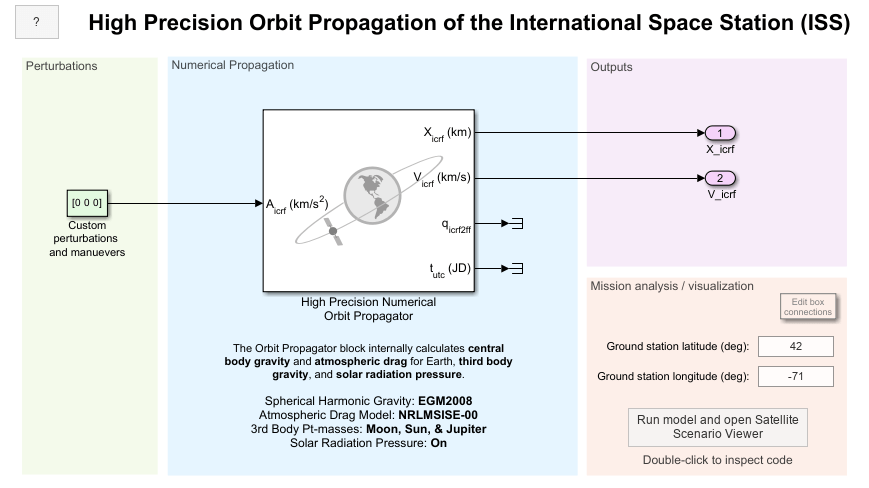Define the path to the Orbit Propagator block in the model.

`iss.blk = mission.mdl + "/High Precision Numerical Orbit Propagator";`

Set spacecraft initial conditions in the Simulink property inspector, or programmatically using `set_param`.

```set_param(iss.blk, ... startDate = string(juliandate(mission.StartDate)), ... stateFormatNum = "ICRF state vector", ... inertialPosition = mat2str(iss.X0_icrf), ... inertialVelocity = mat2str(iss.V0_icrf));```

Set spacecraft physical properties in the Orbit Propagator block.

```set_param(iss.blk, ... mass = string(iss.Mass), ... dragCoeff = string(iss.DragCoeff), ... dragArea = string(iss.DragArea));```

Set spacecraft physical properties in the Perturbations section.

```mission.srpBlk = mission.mdl + "/Solar Radiation Pressure"; set_param(mission.srpBlk + "/SRPCoeff", Value=string(iss.SRPCoeff)); set_param(mission.srpBlk + "/SRPArea", Value=string(iss.SRPArea)); set_param(mission.srpBlk + "/Mass", Value=string(iss.Mass));```

Apply model-level solver setting and save model output port data as a dataset of timetable objects.

```set_param(mission.mdl, ... SolverType = "Variable-step", ... SolverName = "VariableStepAuto", ... RelTol = "1e-12", ... AbsTol = "1e-12", ... StopTime = string(seconds(mission.Duration)), ... SaveOutput = "on", ... OutputSaveName = "yout", ... SaveFormat = "Dataset", ... DatasetSignalFormat = "timetable");```

### Run the Model and Collect Ephemerides

Simulate the model. The Orbit Propagator block is configured to output position and velocity states in the ICRF coordinate frame. The simulation might take several minutes to run due to the strict tolerance settings we applied to the model in the previous section (1e-12).

`mission.SimOutput = sim(mission.mdl);`

Extract position and velocity data from the model output data structure.

```iss.EphPosICRF = mission.SimOutput.yout{1}.Values; iss.EphVelICRF = mission.SimOutput.yout{2}.Values;```

Set the start data from the mission in the `timetable` object to convert the Time row from duration to datetime.

```iss.EphPosICRF.Properties.StartTime = mission.StartDate; iss.EphPosICRF.Time.TimeZone = "UTC"; iss.EphVelICRF.Properties.StartTime = mission.StartDate; iss.EphVelICRF.Time.TimeZone = "UTC";```

### Import Ephemerides into a `satelliteScenario` Object

Create a satellite scenario object.

`scenario = satelliteScenario;`

Add the spacecraft to the `satelliteScenario` as ICRF position and velocity `timetable` objects using the `satellite` method.

```iss.EphPosICRF_m = iss.EphPosICRF; iss.EphPosICRF_m.Data = iss.EphPosICRF.Data*1e3; iss.EphVelICRF_mps = iss.EphVelICRF; iss.EphVelICRF_mps.Data = iss.EphVelICRF.Data*1e3; iss.obj = satellite(scenario, iss.EphPosICRF_m, iss.EphVelICRF_mps, ... CoordinateFrame="inertial", Name="ISS");```

The start and stop time of the scenario are automatically adjusted to reflect the start and stop time of the `timetable` objects.

`disp("Start: " + string(scenario.StartTime)); disp("Stop: " + string(scenario.StopTime));`
```Start: 03-Jan-2022 12:00:00 Stop: 04-Jan-2022 12:00:00 ```

Open the Satellite Scenario Viewer.

`satelliteScenarioViewer(scenario);`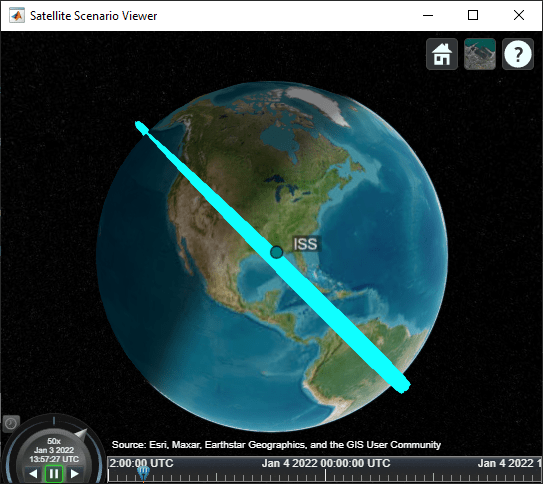### Load the Reference Trajectory and Compare to the Calculated Trajectory

Load ISS trajectory data from Reference .

`iss.RefEphemeris = load("ISS_ephemeris.mat", "pos_eme2000", "vel_eme2000");`

Resample the simulation data to match the 4-minute sample rate of the reference trajectory.

```iss.EphPosICRF = retime(iss.EphPosICRF, iss.RefEphemeris.pos_eme2000.Time); iss.EphVelICRF = retime(iss.EphVelICRF, iss.RefEphemeris.vel_eme2000.Time);```

Plot the position error between the simulation data and the reference trajectory.

```plot(iss.EphPosICRF.Time, iss.EphPosICRF.Data - iss.RefEphemeris.pos_eme2000.Position); title("ISS High Precision Trajectory Position Error"); xlabel("Time"); ylabel("Position Error (km)"); legend(["\DeltaX_{icrf}","\DeltaY_{icrf}","\DeltaZ_{icrf}"], Location="northwest");```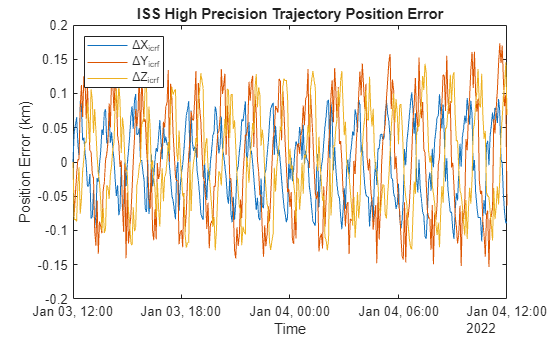The maximum error between the simulation and the reference data over 24 hours is below 0.25km.

```plot(iss.EphPosICRF.Time, vecnorm(iss.EphPosICRF.Data - iss.RefEphemeris.pos_eme2000.Position, 2, 2)); title("ISS High Precision Trajectory Position Error Magnitude"); xlabel("Time"); ylabel("Position Error (km)");```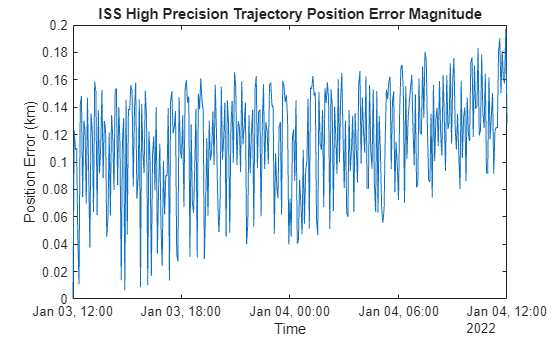### Compare High Precision Trajectory to Analytical Propagation

For comparison, add a new spacecraft to the satellite scenario using Two-Body-Keplerian propagation.

Convert initial position and velocity to orbital elements.

```[iss.OrbEl.SemiMajorAxis, ... iss.OrbEl.Eccentricity, ... iss.OrbEl.Inclination, ... iss.OrbEl.RAAN, ... iss.OrbEl.ArgPeriapsis, ... iss.OrbEl.TrueAnomaly] = ijk2keplerian(iss.X0_icrf*1e3, iss.V0_icrf*1e3);```

Add a new ISS object to the scenario using Two-Body-Keplerian propagation.

```iss.KeplerianOrbit.obj = satellite(scenario, iss.OrbEl.SemiMajorAxis, ... iss.OrbEl.Eccentricity, iss.OrbEl.Inclination, iss.OrbEl.RAAN, ... iss.OrbEl.ArgPeriapsis, iss.OrbEl.TrueAnomaly, ... OrbitPropagator="two-body-keplerian", ... Name="ISS Keplerian"); iss.KeplerianOrbit.obj.MarkerColor = "magenta"; iss.KeplerianOrbit.obj.Orbit.LineColor = "magenta";```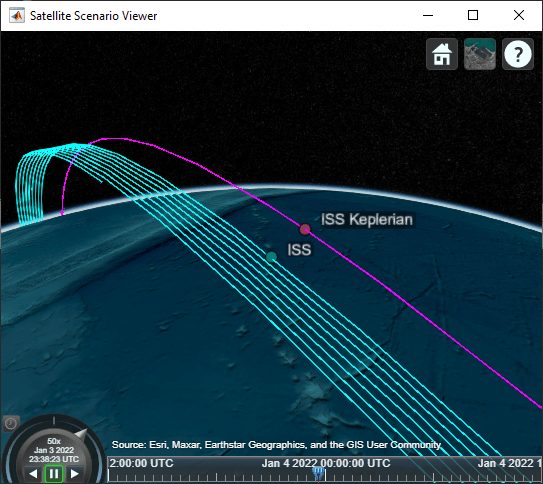For comparison, plot the maximum error using Keplerian two body propagation.

```[iss.KeplerianOrbit.EphPosICRFData, ~, ... iss.KeplerianOrbit.TimeSteps] = states(iss.KeplerianOrbit.obj, CoordinateFrame="inertial"); iss.KeplerianOrbit.EphPosICRF = retime(timetable(iss.KeplerianOrbit.TimeSteps', iss.KeplerianOrbit.EphPosICRFData', VariableNames="Data"), ... iss.RefEphemeris.pos_eme2000.Time); plot(iss.EphPosICRF.Time, iss.KeplerianOrbit.EphPosICRF.Data/1e3 - iss.RefEphemeris.pos_eme2000.Position); title("ISS Two Body Keplerian Position Error"); xlabel("Time"); ylabel("Position Error (km)"); legend(["\DeltaX_{icrf}","\DeltaY_{icrf}","\DeltaZ_{icrf}"], Location="northwest");```The maximum error between the Two-Body-Keplerian propagation and the reference data over 24 hours approaches exceeds 450km, compared to less than 0.25km for the high precision numerical propagation simulation.

```plot(iss.EphPosICRF.Time, vecnorm(iss.KeplerianOrbit.EphPosICRF.Data/1e3 - iss.RefEphemeris.pos_eme2000.Position, 2, 2)); title("ISS Two Body Keplerian Position Error Magnitude"); xlabel("Time"); ylabel("Position Error (km)");```### References

 NASA Open Data Portal - Trajectory Operations and Planning (TOPO), ISS_COORDS_2022-01-03 Public Distribution File - https://data.nasa.gov/Space-Science/ISS_COORDS_2022-01-03/ffwr-gv9k

 Vallado, David. Fundamentals of Astrodynamics and Applications, 4th ed. Hawthorne, CA: Microcosm Press, 2013.